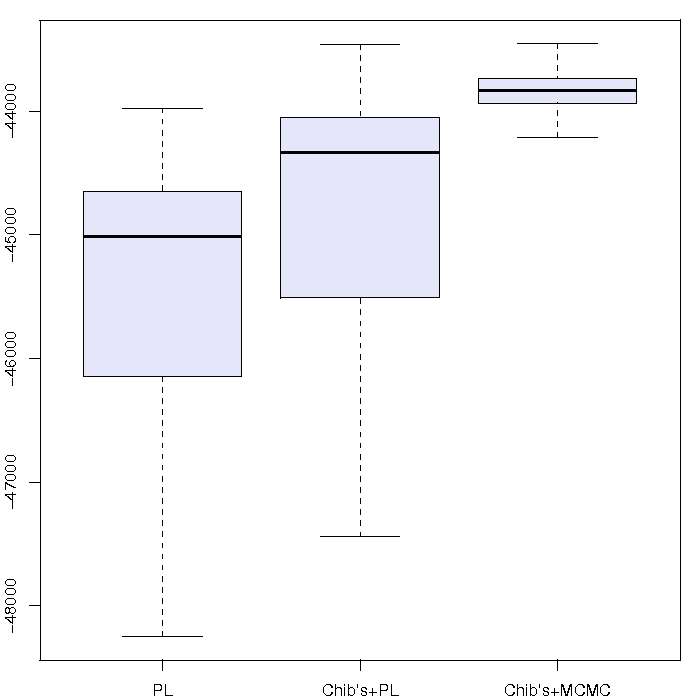Want to share your content on R-bloggers? click here if you have a blog, or here if you don't.

Following the posting on arXiv of the Statistical Science paper of Carvalho et al., and the publication by the same authors in Bayesian Analysis of Particle Learning for general mixtures I noticed on Hedibert Lopes’ website his rejoinder to the discussion of his Valencia 9 paper has been posted. Since the discussion involved several points made by members of the CREST statistics lab (and covered the mixture paper as much as the Valencia 9 paper), I was quite eager to read Hedie’s reply. Unsurprisingly, this rejoinder is however unlikely to modify my reservations about particle learning. The following is a detailed examination of the arguments found in the rejoinder but requires a preliminary reading of the above papers as well as our discussion..“Particle learning based on the product estimate and MCMC based on Chib’s formula produce relatively similar results either for small or large samples”

This statement about the estimation of the marginal likelihood (or the evidence) and the example A that is associated with it thus comes to contradict our (rather intensive) simulation experiment which, as reported in the discussion, concludes to the strong bias in evidence induced by using particle learning, whether or not the product estimator is used. We observed there that there were two levels of degeneracy, one due to the product solution (errors in a product being more prone to go and…multiply) and one due to the particle nature of the sequential method (which does not refresh particles from earlier periods). The above graph is at odds with the one presented in the rejoinder, maybe because we consider 10,000 observations rather than 100. (I also fail to understand how the “Log-predictive (TRUE)” is derived.)

“Black-box sequential importance sampling algorithms and related central limit theorems are of little use in practice.”

Another quote from the rejoinder I do not get. What’s wrong with the central limit theorem?! One major lesson from the central limit theorem is that it provides a scale for the speed of convergence and thus an indicator on the number of particles needed for a given precision level. The authors of the rejoinder then criticise our use of “1000 particles in 5000 dimensional problems” as we “shouldn’t be surprised at all with some of our findings”. I find no trace in the discussion of such a case: we use 10,000 particles in all examples and the target is either the distribution of the 4 mixture parameters, the evidence  or the distribution of a one-dimensional sufficient statistic. Furthermore, these values of n and N are those used in their example D…

“This argument [that the Monte Carlo variance will `blow-up’] is incorrect and extremely misleading.”

This point is central to both the discussion and the rejoinder, as the authors maintain that the inevitable particle degeneracy does not impact the distribution of the sufficient statistics. The argument about using time averages over particle paths rather than sums appears reasonable at first. Actually, taking an empirical average in almost stationary situations should produce an approximately normal distribution. With an asymptotic variance different from 0. (Thanks to the central limit theorem by the way!) However, this is not the main argument used in the discussions. Degeneracy in the particle path means that the early terms in the average are less and less diverse in the sample average. Therefore it is not that surprising that the variance is decreasing to too small a value! As shown in Figure 8 of the discussion, degeneracy due to resampling may induce severe biases in the distribution of empirical averages while giving the impression of less variability. Furthermore, the fact that parameters are simulated [rather than fixed] in the filter means that the  process is not geometrically ergodic, hence that Monte Carlo errors tend to accumulate along iterations, rather than compensate… (This is why the comparison between PL and sampling importance resampling is particularly relevant, because it does not address this accumulation.) The rejoinder also quotes Olsson et al. (2008) for justifying the decrease in the Monte Carlo variance. This is somehow surprising in that (a) Olsson et al. (2008) show that there is degeneracy without a fixed-lag smoothing and (b) they require a geometric forgetting property on the filtering dynamics. In addition, I note that Example E used to illustrate the point about variance reduction is not very appropriate for this issue because the hidden Markov chain is a Gaussian random walk, hence cannot be stationary (a fact noted by the authors). And again a decrease in the “MC error” does not mean a converging algorithm because degeneracy naturally induces empirical variance decrease. (I also fail to see why the “prior” on$(x_t)$ is improper.) The final argument that “PL parameters do not degenerate” is somehow puzzling: by nature, those parameters are simulated from a distribution conditional on the sufficient parameters. So obviously the simulated parameters all differ. But this does not mean that they are marginally distributed from the right distribution.

“MCMC schemes depend upon the not so trivial task of assessing convergence. How long should the burn-in G0 be?”

The rejoinder concludes with recommendations that sound more like a drafted to-do note the authors forgot to remove than an accumulation of true recommendations. It seems to me that the comparison between MCMC and particle filters is not particularly relevant, simply because particle filters apply in [sequential] settings where MCMC cannot be implemented. To try to promote PL over MCM by arguing that MCMC produces dependent draws while having convergence troubles is not needed (besides, PL also produces [unconditional] dependent draws). To advance that the Monte Carlo error for PL is in$C_T/sqrt{N}$ is not more relevant because$C_T$ is exponential in$T$ and because MCMC also has an error in$sqrt{N}$.

﻿

Filed under: R, Statistics, University life Tagged: Bayesian Analysis, convergence diagnostics, degeneracy, discussions, MCMC, mixtures, Monte Carlo Statistical Methods, particle learning, sequential Monte Carlo, Statistical Science, Valencia 9